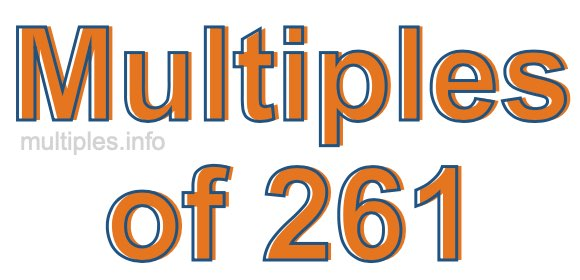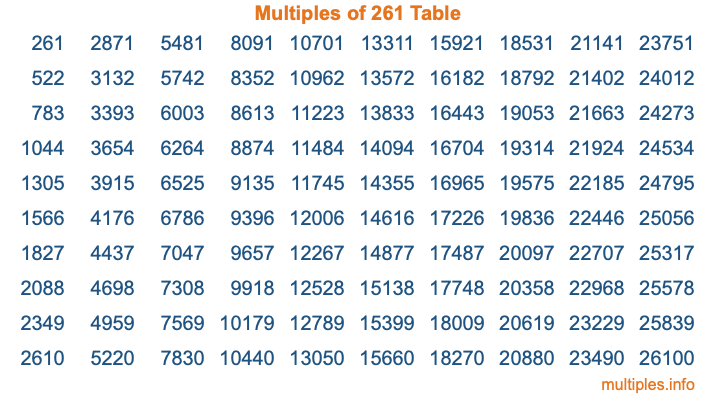Multiples of 261Welcome to the Multiples of 261 page. Here we will first teach you everything you will ever need to know about the multiples of 261, and then give you a study guide summary of everything we taught you to make sure you remember it all. Use this page to look up facts and learn information about the multiples of 261. This page will make you a multiples of two hundred sixty-one expert!

Definition of Multiples of 261
Multiples of 261 are all the numbers that when divided by 261 equal an integer. Each of the multiples of 261 are called a multiple. A multiple of 261 is created by multiplying 261 by an integer.

Therefore, to create a list of multiples of 261, you start with 1 multiplied by 261, then 2 multiplied by 261, then 3 multiplied by 261, and so on for as long as you want. Thus, the list of the first five multiples of 261 is 261, 522, 783, 1044, and 1305. To see a larger list of multiples of 261, see the printable image of Multiples of 261 further down on this page. We also have a category where you can choose any nth multiple of 261.

Multiples of 261 Checker
The Multiples of 261 Checker below checks to see if any number of your choice is a multiple of 261. In other words, it checks to see if there is any number (integer) that when multiplied by 261 will equal your number. To do that, we divide your number by 261. If the the quotient is an integer, then your number is a multiple of 261.

Is  a multiple of 261?

Least Common Multiple of 261 and ...
A Least Common Multiple (LCM) is the lowest multiple that two or more numbers have in common. This is also called the smallest common multiple or lowest common multiple and is useful to know when you are adding our subtracting fractions. Enter one or more numbers below (261 is already entered) to find the LCM.

Check out our LCM Calculator if you need more details about the Least Common Multiple or if you need the LCM for different numbers for adding and subtraction fractions.

nth Multiple of 261
As we stated above, 261 is the first multiple of 261, 522 is the second multiple of 261, 783 is the third multiple of 261, and so on. Enter a number below to find the nth multiple of 261.

th multiple of 261

Multiples of 261 vs Factors of 261
261 is a multiple of 261 and a factor of 261, but that is where the similarities end. All postive multiples of 261 are 261 or greater than 261. All positive factors of 261 are 261 or less than 261.

Below is the beginning list of multiples of 261 and the factors of 261 so you can compare:

Multiples of 261: 261, 522, 783, 1044, 1305, etc.

Factors of 261: 1, 3, 9, 29, 87, 261

As you can see, the multiples of 261 are all the numbers that you can divide by 261 to get a whole number. The factors of 261, on the other hand, are all the whole numbers that you can multiply by another whole number to get 261.

It's also interesting to note that if a number (x) is a factor of 261, then 261 will also be a multiple of that number (x).

Multiples of 261 vs Divisors of 261
The divisors of 261 are all the integers that 261 can be divided by evenly. Below is a list of the divisors of 261.

Divisors of 261: 1, 3, 9, 29, 87, 261

The interesting thing to note here is that if you take any multiple of 261 and divide it by a divisor of 261, you will see that the quotient is an integer.

Multiples of 261 Table
Below is an image of the first 100 multiples of 261 in a table. The table is in chronological order, column by column. The first column has the first ten multiples of 261, the second column has the next ten multiples of 261, and so on.The Multiples of 261 Table is also referred to as the 261 Times Table or Times Table of 261. You are welcome to print out our table for your studies.

Negative Multiples of 261
Although not often discussed or needed in math, it is worth mentioning that you can make a list of negative multiples of 261 by multiplying 261 by -1, then by -2, then by -3, and so on, to get the following list of negative multiples of 261:

-261, -522, -783, -1044, -1305, etc.

Multiples of 261 Summary
Below is a summary of important Multiples of 261 facts that we have discussed on this page. To retain the knowledge on this page, we recommend that you read through the summary and explain to yourself or a study partner why they hold true.

There are an infinite number of multiples of 261.

A multiple of 261 divided by 261 will equal a whole number.

261 divided by a factor of 261 equals a divisor of 261.

The nth multiple of 261 is n times 261.

The largest factor of 261 is equal to the first positive multiple of 261.

261 is a multiple of every factor of 261.

261 is a multiple of 261.

A multiple of 261 divided by a divisor of 261 equals an integer.

261 divided by a divisor of 261 equals a factor of 261.

Any integer times 261 will equal a multiple of 261.

Multiples of a Number
Here you can get the multiples of another number, all with the same attention to detail as we did for multiples of 261 on this page.

Multiples of
Multiples of 262
Did you find our page about multiples of two hundred sixty-one educational? Do you want more knowledge? Check out the multiples of the next number on our list!